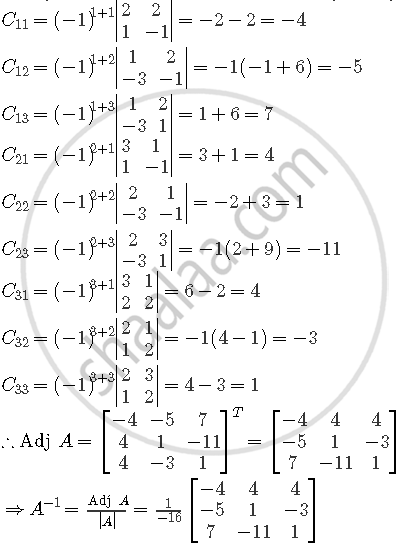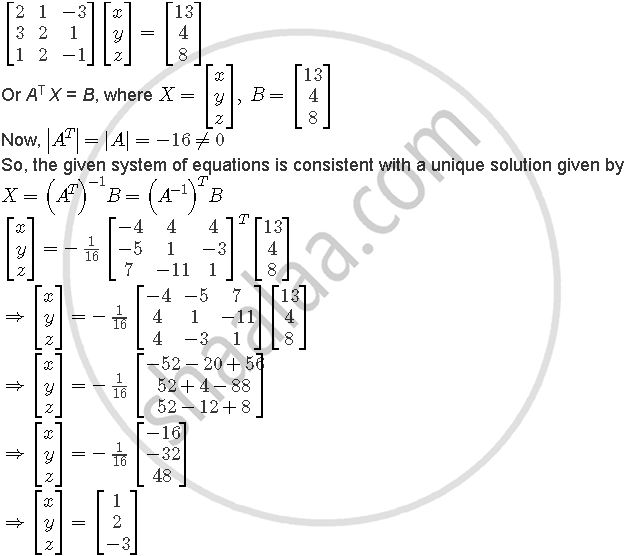# If A = ((2,3,1),(1,2,2),(-3,1,-1)), Find A^(-1) And Hence Solve the System of Equations 2x + Y – 3z = 13, 3x + 2y + Z = 4, X + 2y – Z = 8 - Mathematics

if A = ((2,3,1),(1,2,2),(-3,1,-1)), Find A^(-1) and hence solve the system of equations 2x + y – 3z = 13, 3x + 2y + z = 4, x + 2y – z = 8

#### Solution

We have A = [(2,3,1),(1,2,2),(-3,1,-1)]

". |A| = [(2,3,1),(1,2,2),(-3,1,-1)]

=2(−2 −2) − 3(−1 + 6) +1(1 + 6)

=−8 − 15 + 7=−16 ≠ 0

So, A is invertible.

Let Cij be the co-factors of the elements aij in A[aij]. Then,Now, the given system of equations is expressible asHence, x = 1, = 2 and = −3 is the required solution.

Concept: Invertible Matrices
Is there an error in this question or solution?